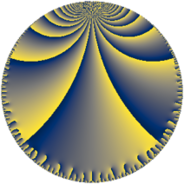# Properties

 Label 48.8.kLevel $48$ Weight $8$ Character orbit 48.k Rep. character $\chi_{48}(11,\cdot)$ Character field $\Q(\zeta_{4})$ Dimension $108$ Newform subspaces $1$ Sturm bound $64$ Trace bound $0$

# Related objects

## Defining parameters

 Level: $$N$$ $$=$$ $$48 = 2^{4} \cdot 3$$ Weight: $$k$$ $$=$$ $$8$$ Character orbit: $$[\chi]$$ $$=$$ 48.k (of order $$4$$ and degree $$2$$) Character conductor: $$\operatorname{cond}(\chi)$$ $$=$$ $$48$$ Character field: $$\Q(i)$$ Newform subspaces: $$1$$ Sturm bound: $$64$$ Trace bound: $$0$$

## Dimensions

The following table gives the dimensions of various subspaces of $$M_{8}(48, [\chi])$$.

Total New Old
Modular forms 116 116 0
Cusp forms 108 108 0
Eisenstein series 8 8 0

## Trace form

 $$108 q - 2 q^{3} - 4 q^{4} + 172 q^{6} - 8 q^{7} + O(q^{10})$$ $$108 q - 2 q^{3} - 4 q^{4} + 172 q^{6} - 8 q^{7} - 6504 q^{10} + 12016 q^{12} - 4 q^{13} - 55280 q^{16} + 21172 q^{18} - 60588 q^{19} - 4376 q^{21} - 212744 q^{22} - 317304 q^{24} + 238042 q^{27} + 219736 q^{28} - 503852 q^{30} - 4 q^{33} - 364856 q^{34} + 867620 q^{36} - 4 q^{37} - 283948 q^{39} + 390392 q^{40} - 3022344 q^{42} + 752844 q^{43} - 156252 q^{45} - 1166824 q^{46} + 358320 q^{48} + 9882508 q^{49} + 1121592 q^{51} - 4458688 q^{52} + 203816 q^{54} + 4191000 q^{55} + 4975544 q^{58} - 4390240 q^{60} - 2279892 q^{61} + 4781432 q^{64} + 1457476 q^{66} + 1552540 q^{67} + 4372 q^{69} - 9366152 q^{70} - 1660280 q^{72} - 886066 q^{75} - 6569272 q^{76} - 13616772 q^{78} - 4 q^{81} + 11560256 q^{82} - 10944176 q^{84} + 7846496 q^{85} - 40789116 q^{87} + 24614816 q^{88} + 25746032 q^{90} - 1590680 q^{91} - 14495396 q^{93} - 6995856 q^{94} - 13927736 q^{96} - 8 q^{97} - 4701076 q^{99} + O(q^{100})$$

## Decomposition of $$S_{8}^{\mathrm{new}}(48, [\chi])$$ into newform subspaces

Label Dim $A$ Field CM Traces $q$-expansion
$a_{2}$ $a_{3}$ $a_{5}$ $a_{7}$
48.8.k.a $108$ $14.994$ None $$0$$ $$-2$$ $$0$$ $$-8$$